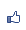TiKz 座標和節點的連結（或是路徑path）
2015/04/10 16:36:13瀏覽1628｜回應0｜推薦0

\coordinate [label=left:{$a$}](a) at (0,0);

\draw (a) circle (0.5);

\node[inner color=white, outer color=orange,inner sep=0.5cm] (b) at (5,2){$b$};

\draw (a)--(b);

\draw (a) controls (1,3) and (5,5)--(b);

\draw (a) -| (b);

\draw (a)|- (b);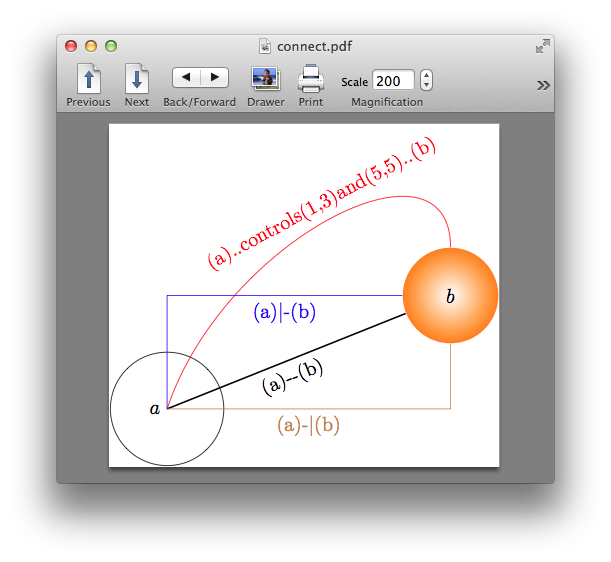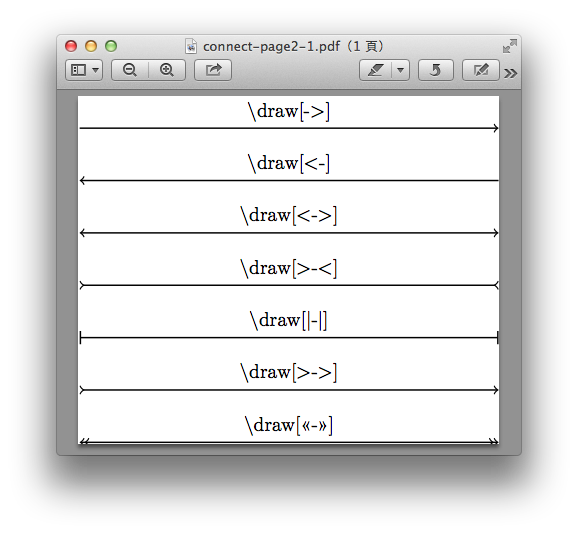\cooridnate [label=left:a](a) at (0,0);

\node[draw,circle,label=right:b] (b) at (6,4);

(a)to(b)，(a)到(b)的直線；

(a)to [out=90, in=90]b，(a)到(b)的曲線，出發方向90度，進入b方向90度。

(a) to [bend right=60]b，(a)到(b)的曲線，出發方向是(a)(b)線段右方60度，進入方向是(a)(b)線段右方60度。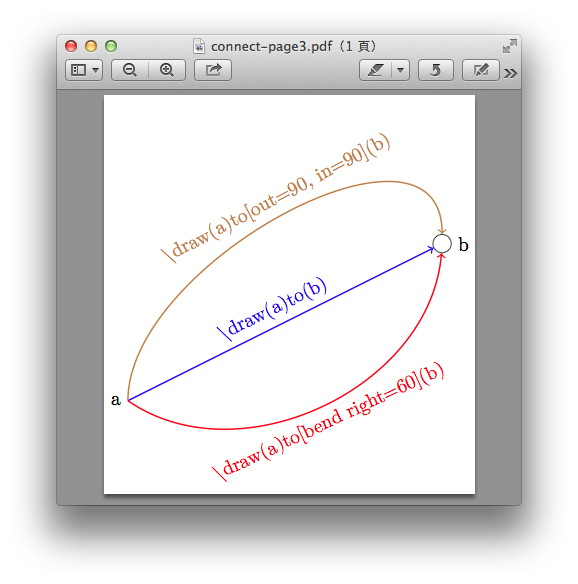\path ... edge (node or coordinate)...; 其本身的意義就是一個路徑。

\path (a) edge (b)

(b) edge[->,red] (c)

(c) edge [-,dotted,black](d)

(d) edge [<-,bend left=""30,blue](a);</p">

\draw (0,0)--(5,3);

\draw (0,0)--node{a}(5,3);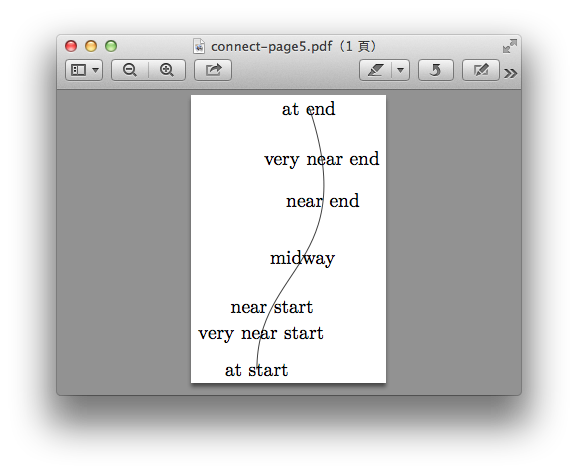\draw (0,0)-- node[near start]{b} node[midway]{a} (5,3);

\draw (0,0)-- node[pos=0.2]{a} (5,3);

\draw (2,0) to[bend left=30] (5,2);

-- node [pos=0.2, left] .. 在0.2位置的右方。

sloped會讓文字旋轉，配合線段該處的斜率。

io: 是表示輸出輸入的node，用梯形。

op: 是表示演算過程的node，用方形。

cn: 是表示判斷過程的node，用菱形。

\begin{tikzpicture}[

io/.style={trapezium, trapezium left angle=70, trapezium right angle=110, fill=magenta!10, draw=magenta}

op/.style={rectangle, fill=orange!10, draw=orange},

cn/.style={diamond, aspect=2, inner sep=2pt, fill=red!10, draw=red},

node distance=5mm, thick]

\node[io] (in) {求$a,b$之最大公因數};

\node[op, below=of in] (div) {$r=a \mod b$};

\node[op, below=of div] (set) {$a=b, \ b=r$};

\node[cn, below=of set] (cond) {$b=0?$};

\node[io, below=of cond] (out) {$a$值是解答};

\path[->]

(in) edge (div)

(div) edge (set)

(set) edge (cond)

(cond) edge node[right]{Yes} (out);

\draw (cond)--node[below]{No} ++(1.5,0) |- (div);

\end{tikzpicture}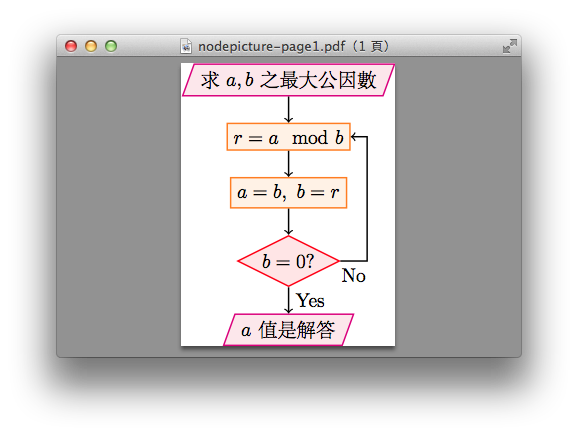( 知識學習科學百科 )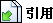引用網址：https://classic-blog.udn.com/article/trackback.jsp?uid=paraquat&aid=22113130